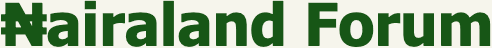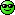#Welcome, Guest: Join Nairaland / LOGIN! / Trending / Recent / New
Stats: 2,775,451 members, 6,611,625 topics. Date: Wednesday, 01 December 2021 at 12:57 PM

## Solve This Mathematics Problem - Education - Nairaland

 Solve This Mathematics Problem by Johnpaul01: 12:08pm On Jan 17, 2017 Help me out, its an assignment and am getting confused find the positive square root of 35-12√6 Re: Solve This Mathematics Problem by laperson: 4:48pm On Jan 17, 2017 The positive square root is 3√3-2√2I will send the solution to you ASAPRegards Re: Solve This Mathematics Problem by laperson: 5:03pm On Jan 17, 2017 I couldn't upload the solution, don't understand what is happening-I didn't exceed the 200Kb limit for files. I will try to type the solution for you but that might take some time.Regards Re: Solve This Mathematics Problem by laperson: 5:21pm On Jan 17, 2017 Solution:Let the square root of 35-12√6 be represented by √a - √b Therefore, the 35-12√6 = (√a - √b)square= a+b-2√ab comparing the equation and quantities above, we can clearly observe thata+b=35----------- (1)2√ab=12√6√ab=6√6square bothsidesab=216------------(2)Solving equations (1) and (2) simultaneously,let a=35-b---------(3)put (3) into (2), we obtain(35-b)(b)=216exploring further36b-b[/sup]2=216rearranging in quadratic form will giveb[sup]2-35b+216=0solving by factorisation methodb[sup][/sup]-27b-8b+216=0b(b-27)-8(b-27)=0b=8 or 27However, when b=8, a=35-b=35-8=27 (a=27 and b=case 1 when b=27, a=35-b=35-27=8 (a=8 and b=27) case 2Let us now explore case 1: a=27 and b=8Note that the square root= √a - √b =√27 - √8= 3√3 - 2√2...This is positive because 3√3 - 2√2>0but if you consider case 2: a=8 and b=27The square root becomes the reverse i.e 2√2 - 3√3...This is negative because 2√2 - 3√3<0Therefore, the positive square root is 3√3 - 2√2 and it is obtained when a=27 and b=8 in case 1 aboveRegards Re: Solve This Mathematics Problem by Johnpaul01: 7:48pm On Jan 17, 2017 laperson:Solution:Let the square root of 35-12√6 be represented by √a - √b Therefore, the 35-12√6 = (√a - √b)square= a+b-2√ab comparing the equation and quantities above, we can clearly observe thata+b=35----------- (1)2√ab=12√6√ab=6√6square bothsidesab=216------------(2)Solving equations (1) and (2) simultaneously,let a=35-b---------(3)put (3) into (2), we obtain(35-b)(b)=216exploring further36b-b[/sup]2=216rearranging in quadratic form will giveb[sup]2-35b+216=0solving by factorisation methodb[sup][/sup]-27b-8b+216=0b(b-27)-8(b-27)=0b=8 or 27However, when b=8, a=35-b=35-8=27 (a=27 and b=case 1 when b=27, a=35-b=35-27=8 (a=8 and b=27) case 2Let us now explore case 1: a=27 and b=8Note that the square root= √a - √b =√27 - √8= 3√3 - 2√2...This is positive because 3√3 - 2√2>0but if you consider case 2: a=8 and b=27The square root becomes the reverse i.e 2√2 - 3√3...This is negative because 2√2 - 3√3<0Therefore, the positive square root is 3√3 - 2√2 and it is obtained when a=27 and b=8 in case 1 aboveRegardsThanks bro, God bless you 1 Like Re: Solve This Mathematics Problem by Johnpaul01: 8:41am On Jan 29, 2017 pls I need solutions to these1. solve 2x-3y=1,x²+xy-4y²=22. resolve into partial fractiona. x²/(x+1)²b. 4x+7/(x²+3)(x²+5)c. 2x³+3x²+7/(x+1)²(x²+1)3. When ax³+bx²+cx-4 is divided by x+2. the remainder is double that is obtained when the expression is divided by x+1. show that C can have any value, and find b in term of a.4. solve x-1/(x-2)(x+1)<0the mathematicians in d house please help me solve d ones you can Re: Solve This Mathematics Problem by combine: 1:33pm On Mar 03, 2017 Cheap stuff Re: Solve This Mathematics Problem by Johnpaul01: 12:55pm On Mar 04, 2017 combine:Cheap stuff After we submitted it already??